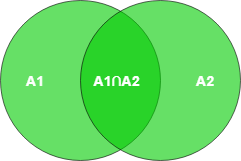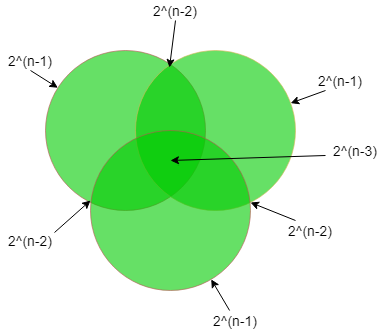GeeksforGeeks App
Open AppBrowser
Continue

# Number of Possible Super Keys in DBMS

Any set of attributes of a table that can uniquely identify all the tuples of that table is known as a Super key. It’s different from the primary and candidate keys in the sense that only the minimal superkeys are the candidate/primary keys.

`Prerequisite - Relational Model Introduction and Codd Rules `

This means that from a super key when we remove all the attributes that are unnecessary for its uniqueness, only then it becomes a primary/candidate key. So, in essence, all primary/candidate keys are super keys but not all super keys are primary/candidate keys. By the formal definition of a Relation(Table), we know that the tuples of a relation are all unique. So, the set of all attributes itself is a super key.

Counting the possible number of super keys for a table is a common question for GATE. The examples below will demonstrate all possible types of questions on this topic.

Example-1: Let a Relation R have attributes {a1,a2,a3} and a1 is the candidate key. Then how many super keys are possible?

Here, any superset of a1 is the super key.
Super keys are = {a1, a1 a2, a1 a3, a1 a2 a3}
Thus we see that 4 Super keys are possible in this case.

In general, if we have ‘N’ attributes with one candidate key then the number of possible superkeys is 2(N – 1)

Example-2 : Let a Relation R have attributes {a1, a2, a3,…,an}. Find Super key of R.

Maximum Super keys = 2n – 1.

Proof: There are n attributes in R. So the total number of possible subsets/combination of attributes of R is 2n

Now to be a Super key, there should be at least one attribute present i.e. the NULL set or the set with no attribute can’t be a super key.

So, maximum possible number of Super keys of R = 2n – 1.

Example-3: Let a Relation R have attributes {a1, a2, a3,…, an} and the candidate key is “a1 a2 a3” then the possible number of super keys?

Following the previous formula, we have 3 attributes instead of one. So, here the number of possible super keys is 2(N-3)

Example-4: Let a Relation R have attributes {a1, a2, a3,…, an} and the candidate keys are “a1”, “a2” then the possible number of super keys?

This problem now is slightly different since we now have two different candidate keys instead of only one. Tackling problems like these is shown in the diagram below:`→ |A1 ∪ A2| = |A1| + |A2| - |A1 ∩ A2| `

= (super keys possible with candidate key A1) + (super keys possible with candidate key A2) – (common superkeys from both A1 and A2)

`= 2(n-1) + 2(n-1) - 2(n-2)  `

Example-5: Let a Relation R have attributes {a1, a2, a3,…, an} and the candidate keys are “a1”, “a2 a3” then the possible number of super keys?

Super keys of (a1) + Super keys of (a2 a3) – Super keys of (a1 a2 a3)

`⇒ 2(n - 1) + 2(n - 2) - 2(n - 3) `

Example-6: Let a Relation R have attributes {a1, a2, a3,…, an} and the candidate keys are “a1 a2”, “a3 a4” then the possible number of super keys?

Super keys of(a1 a2) + Super keys of(a3 a4) – Super keys of(a1 a2 a3 a4)

`⇒ 2(n - 2) + 2(n - 2) - 2(n - 4)  `

Example-7: Let a Relation R have attributes {a1, a2, a3,…, an} and the candidate keys are “a1 a2”, “a1 a3” then the possible number of super keys?

Super keys of (a1 a2) + Super keys of (a1 a3) – Super keys of(a1 a2 a3)

`⇒ 2(n - 2) + 2(n - 2) - 2(n - 3)  `

Example-8 : Let a Relation R have attributes {a1, a2, a3,…,an} and the candidate keys are “a1”, “a2”, “a3” then the possible number of super keys?

In this question, we have 3 different candidate keys. Tackling problems like these are shown in the diagram below.→ |A1 ∪ A2 ∪ A3| = |A1| + |A2| + |A3| – |A1 ∩ A2| – |A1 ∩ A3| – |A2 ∩ A3| + |A1 ∩ A2 ∩ A3|

= (super keys possible with candidate key A1) + (super keys possible with candidate key A2) + (super keys possible with candidate key A3) – (common super keys from both A1 and A2) – (common super keys from both A1 and A3) – (common super keys from both A2 and A3) + (common super keys from both       A1, A2, and A3)

= 2(n-1) + 2(n-1) + 2(n-1) – 2(n-2) – 2(n-2) – 2(n-2) + 2(n-3)

Example-9: A relation R (A, B, C, D, E, F, G, H)and set of functional dependencies are

```CH → G,
A → BC,
B → CFH,
E → A,
F → EG ```

Then how many possible super keys are present?

Step 1:- First of all, we have to find what the candidate keys are:-
as we can see in the given functional dependency D is missing but in relation, D is given so D must be a prime attribute of the Candidate key.

`A+ = E+ = B+ = F+ = all attributes of a relation except D `

So, Candidate keys are = AD, BD, ED, FD

Step 2:-Find super keys due to a single candidate key there is a two possibilities of attribute either we select or not hence there will be 2 chances so,

`A_ _D_ _ _ _ = _ B_ D_ _ _ _ = _ _ _ DE _ _ _ = _ _ _ D_F_ _ = 26 `

Step 3:-Find superkeys due to a combination of two Candidate Keys. So,

`n(AD ∩ BD) = n(AD ∩ ED) = n(AD ∩ FD) = n(BD ∩ ED) = n(BD ∩ FD) = n(ED ∩ FD) = 25 `

Step 4:-Find super keys due to a combination of 3 Candidate Keys
So,
n(AD ∩ BD ∩ ED) = n(AD ∩ ED ∩ FD) = n(ED ∩ BD ∩ FD) = n(BD ∩ FD ∩ AD) = 24

Step 5:-Find super keys due to all. So,

`n(AD ∩ BD ∩ ED ∩ FD) = AB_DEF_ _ = 23 `

So, According to the inclusion-exclusion principle :-

|W ∪ X ∪ Y ∪ Z| = |W| + |X| + |Y| + |Z| – |W ∩ X| – |W ∩ Y| – |W ∩ Z| – |X ∩Y| – |X ∩ Z| – |Y ∩ Z| + |W ∩ X ∩ Y| + |W ∩ X ∩ Z| + |W ∩ Y ? Z| + |X ∩ Y ∩ Z|                   – |W ∩ X ∩ Y ∩ Z|

# Super keys = 4 * 26 – 6 * 25 + 4 * 24 – 23 = 120

So the number of super keys is 120.

Example 10 : Let a Relation R have attributes {a1,a2,a3______ an} and {a1a2a3____ak} as the candidate key where k<=n. Then how many super keys are possible?
The possible number of superkeys is 2(n-k).

Example 11: Let a relation R have attributes {a1,a2,a3______ an} such that any k of the attributes at a time determines all other attributes. Find the value of k such that the number of candidate keys in the relation will be maximum.

Any k attributes at a time constitute one candidate key. These k attributes are randomly chosen from the n attributes. So for some k, the possible no of candidate keys is nCk,i.e, n!/(n-k)!k!. For the number of members to be maximum k must be ⌊n/2⌋ so that nCk is the maximum for that value.

My Personal Notes arrow_drop_up